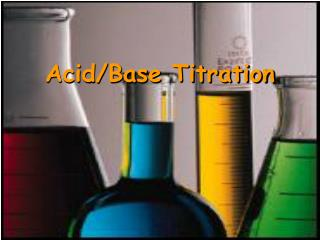DownloadDownload PresentationAcid/Base Titration

# Acid/Base Titration

Télécharger la présentation## Acid/Base Titration

- - - - - - - - - - - - - - - - - - - - - - - - - - - E N D - - - - - - - - - - - - - - - - - - - - - - - - - - -
##### Presentation Transcript

1. Acid/Base Titration

2. Acid–Base Titration • The concentration of a weak acid or a weak base in water is difficult – if not impossible – to measure directly. • But we can calculate the concentration from the results of titration.

3. Acid–Base Titration • A titration is a carefully controlled neutralization rxn. • To run a titration on a soln of unknown conc of an acid or a base, you need a 2nd soln called a standard soln. • A standard soln contains acid or base in a known conc

4. Acid–Base Titration • If the unknown soln is an acid, the standard soln is a base • And vice a versa • Another substance involved in a titration is an indicator. • which is a substance that changes color at a certain pH

5. Acid–Base Titration • Most of the time we use the common indicator Phenolphthalein. • Phenolphthalein is clear in acid • Light pink in neutral • Dark pink in base

6. Procedure for Titration • the standard is slowly added to the unknown soln • the 2 solns mix, the acid in one neutralizes the base in the other • Eventually, enough standard soln is added to neutralize all of the acid or base in the unknown soln.

7. 1 2 4 3

8. Procedure for Titration • The point at which this occurs is called the equivalence point. • [H30+] = [OH-] • The point at which the indicator changes color is called the end point of the titration.

9. Procedure for Titration • If the indicator is chosen correctly the end point is very close to the equivalence point. • Therefore, at approximately the end pt of a titration the total mols of H+ donated by the acid is equal to the total mols of H+ accepted by the base.

10. Total moles of H+ from the acid Total moles of OH- from the base = Procedure for Titration • For Arrhenius Acids and Bases we can say: • This is key to solving titration calcs

11. AH A-

12. AH A-

13. AH A-

14. AH A-

15. AH A-

16. AH A-

17. A- AH

18. A- AH

19. A- AH

20. A- AH

21. A- AH

22. Weak Acid/Strong Base Titration A solution that is 0.10 M CH3COOH is titrated with 0.10 M NaOH Endpoint is above pH 7

23. Strong Acid/Strong Base Titration Endpoint is at pH 7 A solution that is 0.10 M HCl is titrated with 0.10 M NaOH

24. Strong Acid/Strong Base Titration A solution that is 0.10 M NaOH is titrated with 0.10 M HCl Endpoint is at pH 7 It is important to recognize that titration curves are not always increasing from left to right.

25. Strong Acid/Weak Base Titration A solution that is 0.10 M HCl is titrated with 0.10 M NH3 Endpoint is below pH 7

26. Sample Problem Solns of NaOH are used to unclog drains. A 43 ml volume of NaOH was titrated with 32 ml of .100M HCl. What is the molarity of the NaOH soln?

27. Analyze: • At the equivalence pt, the added acid has completely neutralized the unknown base. • It took 32 ml of acid to neutralize 43 ml of base • We know that mols of base = mols of acid, at the equiv pt

28. Solve: • moles of acid used: • (.100mols/L)(.032L)= .0032mol of acid • mols of acid = mols of base • .0032 mol base used in rxn. • Molarity of NaOH • .0032mol/.043L = .074M